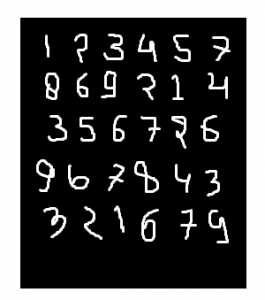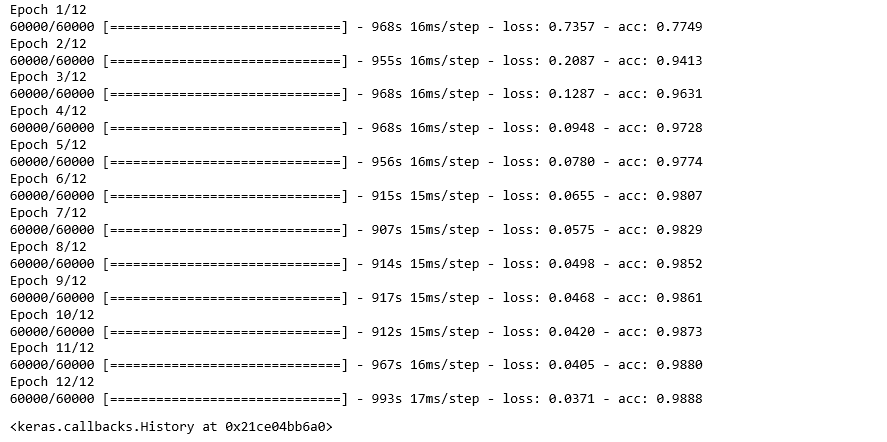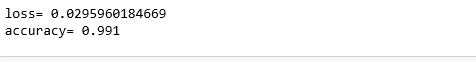# Applying Convolutional Neural Network on mnist dataset

CNN is basically a model known to be Convolutional Neural Network and in the recent time it has gained a lot of popularity because of it’s usefullness. CNN uses multilayer perceptrons to do computational works. CNNs use relatively little pre-processing compared to other image classification algorithms. This means the network learns through filters that in traditional algorithms were hand-engineered. So, for image processing task CNNs are the best-suited option.

MNIST dataset:
mnist dataset is a dataset of handwritten images as shown below in image.We can get 99.06% accuracy by using CNN(Convolutionary neural Network) with functional model. The reason of using functional model is maintaining easiness while connecting the layers.

• #### Firstly, include all necessary libraries

 `import` `numpy as np ` `import` `keras  ` `from` `keras.datasets ``import` `mnist ` `from` `keras.models ``import` `Model ` `from` `keras.layers ``import` `Dense, ``Input` `from` `keras.layers ``import` `Conv2D, MaxPooling2D, Dropout, Flatten ` `from` `keras ``import` `backend as k `

• #### Create the train data and test data

Test data: Used for testing the model that how are model has been trained.
Train data: Used to train our model.

 `(x_train, y_train), (x_test, y_test) ``=` `mnist.load_data() `

While proceeding further, img_rows and img_cols are used as the image dimensions. In mnist dataset, it is 28 and 28. We also need to check the data format i.e. ‘channels_first’ or ‘channels_last’. In CNN, we can normalize data before hands such that large terms of the calculations can be reduced to smaller terms. Like, we can normalize the x_train and x_test data by dividing it with 255.

Checking data-format:

 `img_rows, img_cols``=``28``, ``28` ` `  `if` `k.image_data_format() ``=``=` `'channels_first'``: ` `   ``x_train ``=` `x_train.reshape(x_train.shape[``0``], ``1``, img_rows, img_cols) ` `   ``x_test ``=` `x_test.reshape(x_test.shape[``0``], ``1``, img_rows, img_cols) ` `   ``inpx ``=` `(``1``, img_rows, img_cols) ` ` `  `else``: ` `   ``x_train ``=` `x_train.reshape(x_train.shape[``0``], img_rows, img_cols, ``1``) ` `   ``x_test ``=` `x_test.reshape(x_test.shape[``0``], img_rows, img_cols, ``1``) ` `   ``inpx ``=` `(img_rows, img_cols, ``1``) ` ` `  `x_train ``=` `x_train.astype(``'float32'``) ` `x_test ``=` `x_test.astype(``'float32'``) ` `x_train ``/``=` `255` `x_test ``/``=` `255`

• #### Description of the output classes:

Since output of the model can comprise of any of the digits between 0 to 9.so, we need 10 classes in output. To make output for 10 classes, use `keras.utils.to_categorical` function, which will provide with the 10 columns. Out of these 10 columns only one value will be one and rest 9 will be zero and this one value of the output will denote the class of the digit.

 `y_train ``=` `keras.utils.to_categorical(y_train) ` `y_test ``=` `keras.utils.to_categorical(y_test) `

Now, dataset is ready so let’s move towards the cnn model :

 `inpx ``=` `Input``(shape``=``inpx) ` `layer1 ``=` `Conv2D(``32``, kernel_size``=``(``3``, ``3``), activation``=``'relu'``)(inpx) ` `layer2 ``=` `Conv2D(``64``, (``3``, ``3``), activation``=``'relu'``)(layer1) ` `layer3 ``=` `MaxPooling2D(pool_size``=``(``3``, ``3``))(layer2) ` `layer4 ``=` `Dropout(``0.5``)(layer3) ` `layer5 ``=` `Flatten()(layer4) ` `layer6 ``=` `Dense(``250``, activation``=``'sigmoid'``)(layer5) ` `layer7 ``=` `Dense(``10``, activation``=``'softmax'``)(layer6) `

• Explanation of the working of each layer in CNN model:

layer1 is Conv2d layer which convolves the image using 32 filters each of size (3*3).
layer2 is again a Conv2D layer which is also used to convolve the image and is using 64 filters each of size (3*3).
layer3 is MaxPooling2D layer which picks the max value out of a matrix of size (3*3).
layer4 is showing Dropout at a rate of 0.5.
layer5 is flattening the output obtained from layer4 and this flatten output is passed to layer6.
layer6 is a hidden layer of neural network containng 250 neurons.
layer7 is the output layer having 10 neurons for 10 classes of output that is using the softmax function.

• Calling compile and fit function:

 `model ``=` `Model([inpx], layer7) ` `model.``compile``(optimizer``=``keras.optimizers.Adadelta(), ` `              ``loss``=``keras.losses.categorical_crossentropy, ` `              ``metrics``=``[``'accuracy'``]) ` ` `  `model.fit(x_train, y_train, epochs``=``12``, batch_size``=``500``) `Firstly, we made an object of the model as shown in the above given lines, where [inpx] is the input in the model and layer7 is the output of the model. We compiled the model using required optimizer, loss function and printed the accuracy and at the last model.fit was called along with parameters like x_train(means image vectors), y_train(means the label), number of epochs and the batch size. Using fit function x_train, y_train dataset is fed to model in a particular batch size.

• Evaluate function:
model.evaluate provides the score for the test data i.e. provided the test data to the model. Now, model will predict class of the data and predicted class will be matched with y_test label to give us the accuracy.

 `score ``=` `model.evaluate(x_test, y_test, verbose``=``0``) ` `print``(``'loss='``, score[``0``]) ` `print``(``'accuracy='``, score[``1``]) `

Output:Attention reader! Don’t stop learning now. Get hold of all the important CS Theory concepts for SDE interviews with the CS Theory Course at a student-friendly price and become industry ready.

My Personal Notes arrow_drop_upCheck out this Author's contributed articles.

If you like GeeksforGeeks and would like to contribute, you can also write an article using contribute.geeksforgeeks.org or mail your article to contribute@geeksforgeeks.org. See your article appearing on the GeeksforGeeks main page and help other Geeks.

Please Improve this article if you find anything incorrect by clicking on the "Improve Article" button below.

Improved By : nidhi_biet